# dplyr & tidyverse for data processing

Overview
Questions:
• How can I load tabular data into R?

• How can I slice and dice the data to ask questions?

Objectives:
• Read data with the built-in `read.csv`

• Read data with dplyr’s `read_csv`

• Use dplyr and tidyverse functions to cleanup data.

Requirements:
Time estimation: 1 hour
Supporting Materials:
Last modification: Oct 23, 2023
purl PURL: https://gxy.io/GTN:T00104
version Revision: 8
Best viewed in RStudio

This tutorial is available as an RMarkdown file and best viewed in RStudio! You can load this notebook in RStudio on one of the UseGalaxy.* servers

Launching the notebook in RStudio in Galaxy

1. Instructions to Launch RStudio
2. Access the R console in RStudio (bottom left quarter of the screen)
3. Run the following code:
``````download.file("https://training.galaxyproject.org/training-material/topics/data-science/tutorials/r-dplyr/data-science-r-dplyr.Rmd", "data-science-r-dplyr.Rmd")
``````
4. Double click the RMarkdown document that appears in the list of files on the right.

1. Right click this link: tutorial.Rmd

Alternative Formats

1. This tutorial is also available as a Jupyter Notebook (With Solutions), Jupyter Notebook (Without Solutions)
Hands-on: Learning with RMarkdown in RStudio

Learning with RMarkdown is a bit different than you might be used to. Instead of copying and pasting code from the GTN into a document you’ll instead be able to run the code directly as it was written, inside RStudio! You can now focus just on the code and reading within RStudio.

1. Load the notebook if you have not already, following the tip box at the top of the tutorial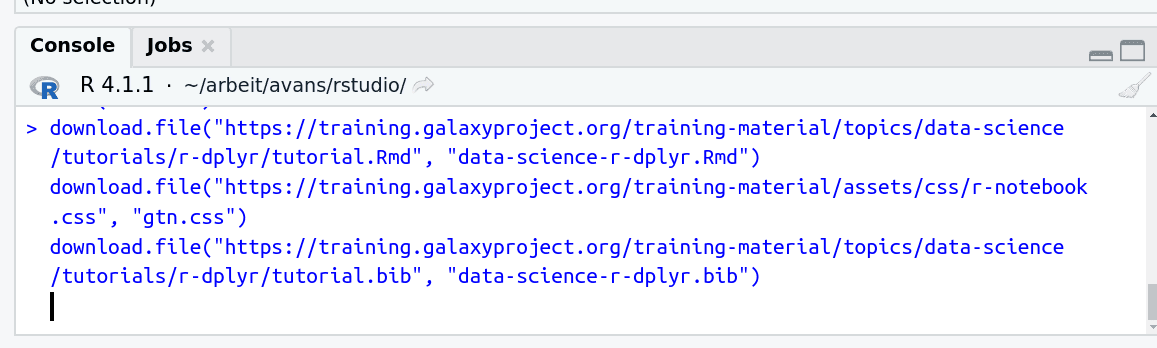2. Open it by clicking on the `.Rmd` file in the file browser (bottom right)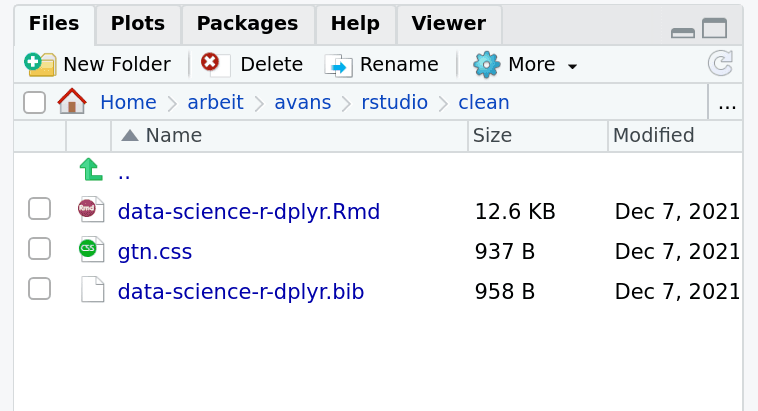3. The RMarkdown document will appear in the document viewer (top left)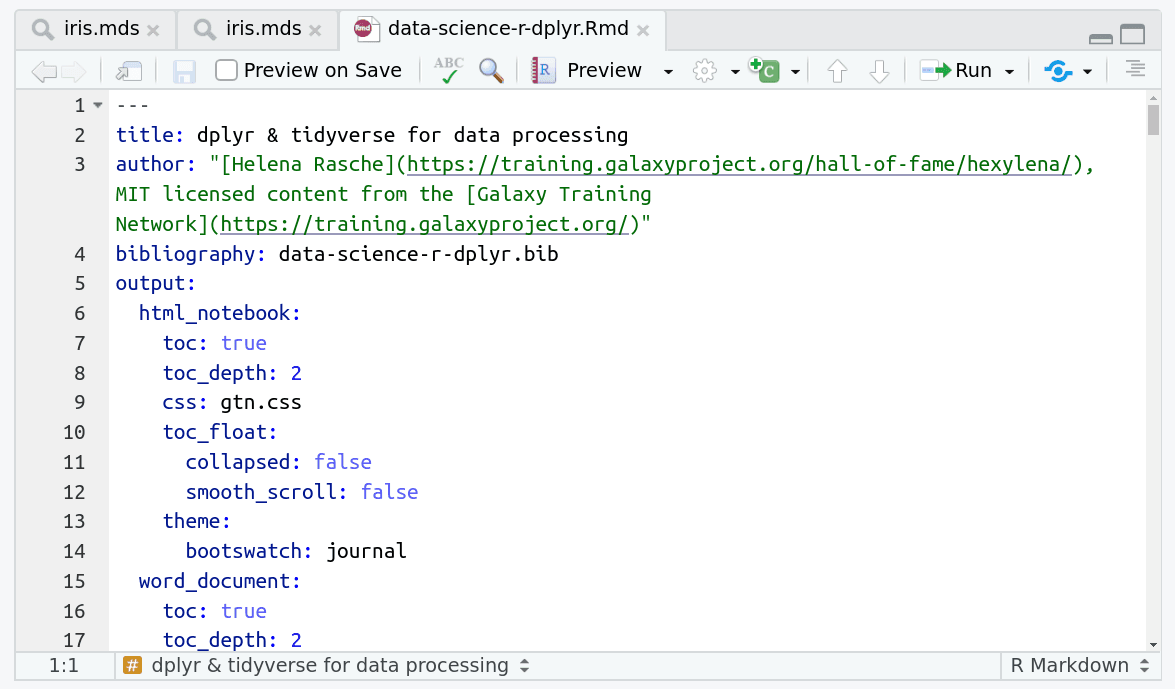You’re now ready to view the RMarkdown notebook! Each notebook starts with a lot of metadata about how to build the notebook for viewing, but you can ignore this for now and scroll down to the content of the tutorial.

You can switch to the visual mode which is way easier to read - just click on the gear icon and select `Use Visual Editor`.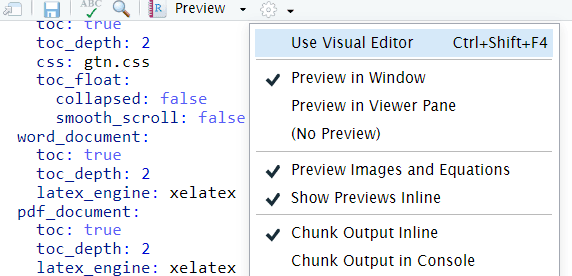You’ll see codeblocks scattered throughout the text, and these are all runnable snippets that appear like this in the document: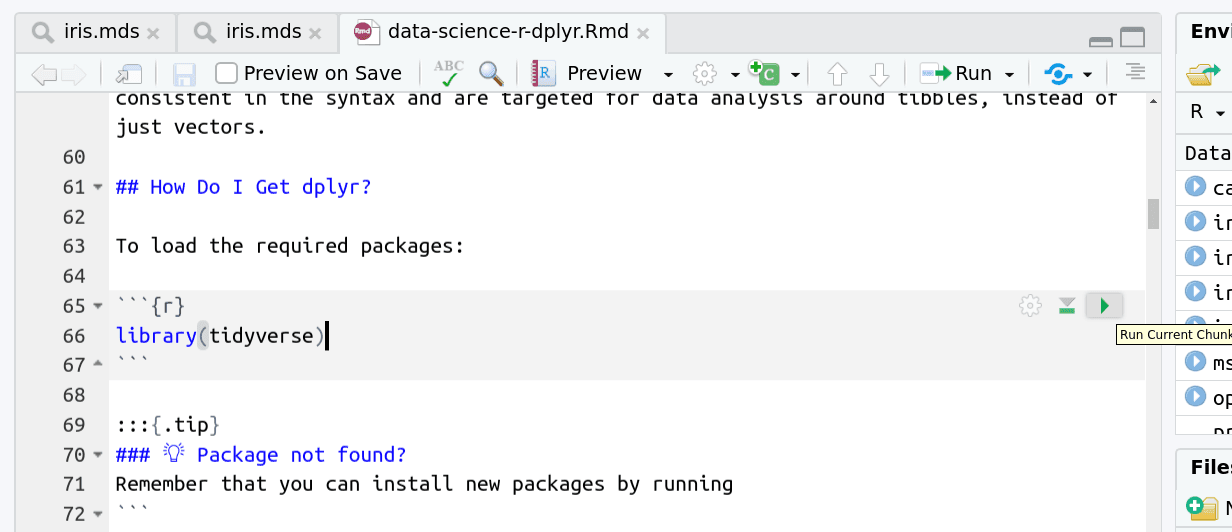And you have a few options for how to run them:

1. Click the green arrow
2. ctrl+enter
3. Using the menu at the top to run all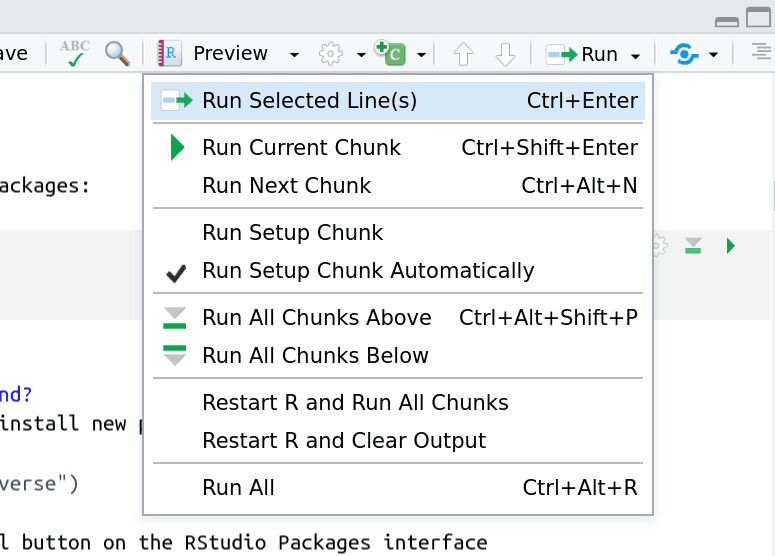When you run cells, the output will appear below in the Console. RStudio essentially copies the code from the RMarkdown document, to the console, and runs it, just as if you had typed it out yourself!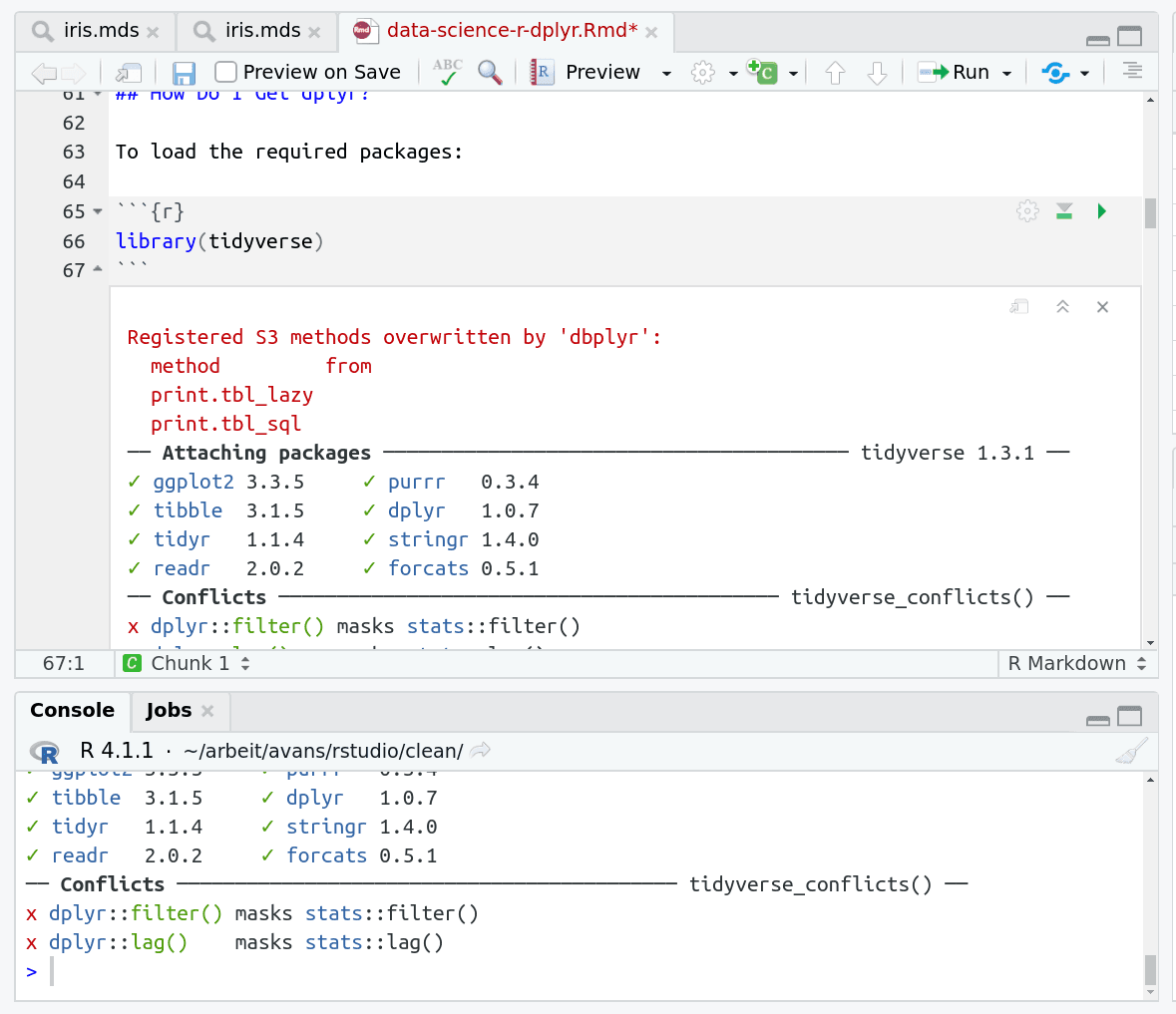One of the best features of RMarkdown documents is that they include a very nice table browser which makes previewing results a lot easier! Instead of needing to use `head` every time to preview the result, you get an interactive table browser for any step which outputs a table.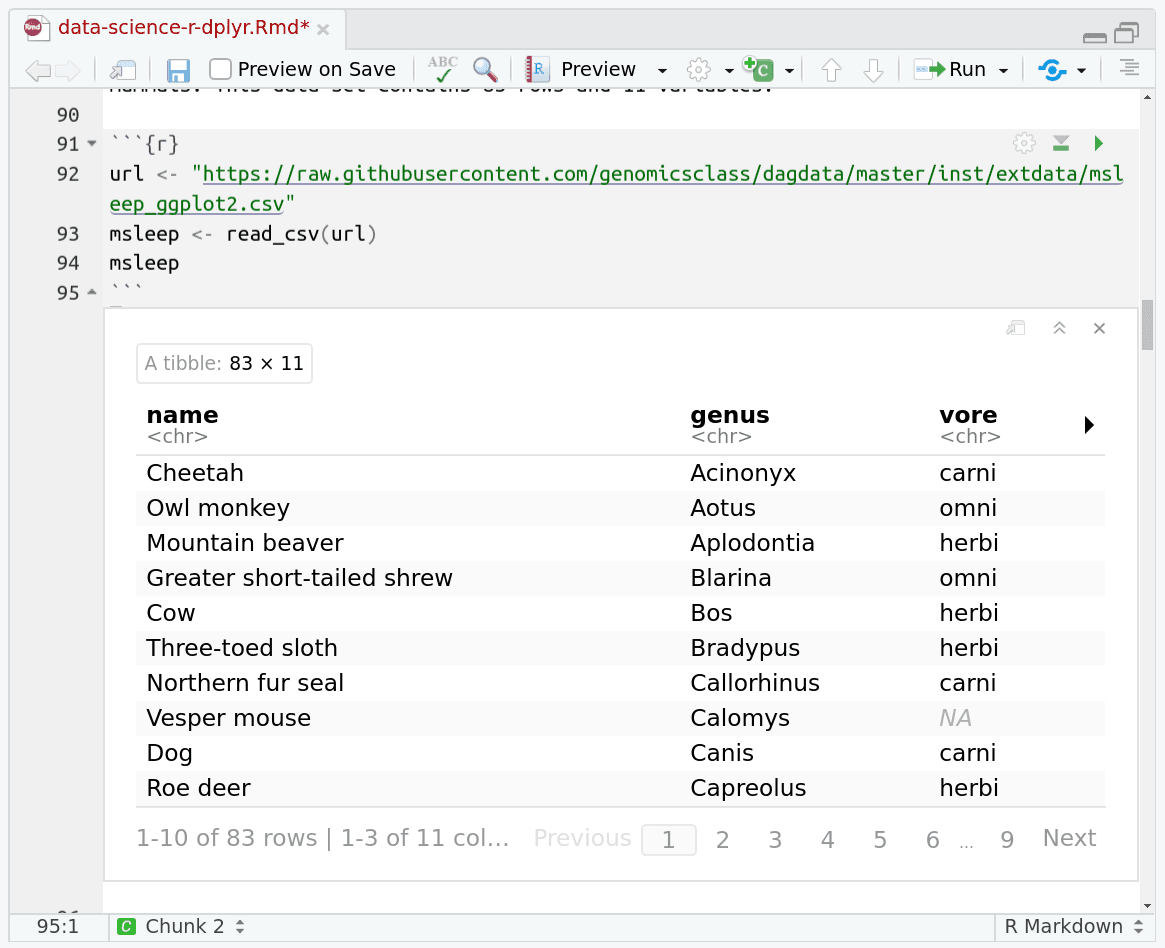dplyr (Wickham et al. 2021) is a powerful R-package to transform and summarize tabular data with rows and columns. It is part of a group of packages (including `ggplot2`) called the `tidyverse` (Wickham et al. 2019), a collection of packages for data processing and visualisation. For further exploration please see the dplyr package vignette: Introduction to dplyr

Comment

This tutorial is significantly based on GenomicsClass/labs.

Agenda

In this tutorial, we will cover:

## Why Is It Useful?

The package contains a set of functions (or “verbs”) that perform common data manipulation operations such as filtering for rows, selecting specific columns, re-ordering rows, adding new columns and summarizing data.

In addition, dplyr contains a useful function to perform another common task which is the “split-apply-combine” concept. We will discuss that in a little bit.

## How Does It Compare To Using Base Functions R?

If you are familiar with R, you are probably familiar with base R functions such as split(), subset(), apply(), sapply(), lapply(), tapply() and aggregate(). Compared to base functions in R, the functions in dplyr are easier to work with, are more consistent in the syntax and are targeted for data analysis around tibbles, instead of just vectors.

## How Do I Get dplyr?

``````library(tidyverse)
``````

Remember that you can install new packages by running

``````install.packages("tidyverse")
``````

Or by using the Install button on the RStudio Packages interface

Here we’ve imported the entire suite of tidyverse packages. We’ll specifically be using:

Package Use
`readr` This provides the `read_csv` function which is identical to `read.csv` except it returns a tibble
`dplyr` All of the useful functions we’ll be covering are part of dplyr
`magrittr` A dependency of `dplyr` that provides the `%>%` operator
`ggplot2` The famous plotting library which we’ll use at the very end to plot our aggregated data.

# Data: Mammals Sleep

The msleep (mammals sleep) data set contains the sleep times and weights for a set of mammals. This data set contains 83 rows and 11 variables.

``````url <- "https://raw.githubusercontent.com/genomicsclass/dagdata/master/inst/extdata/msleep_ggplot2.csv"
``````

The columns (in order) correspond to the following:

column name Description
name common name
genus taxonomic rank
vore carnivore, omnivore or herbivore?
order taxonomic rank
conservation the conservation status of the mammal
sleep_total total amount of sleep, in hours
sleep_rem rem sleep, in hours
sleep_cycle length of sleep cycle, in hours
awake amount of time spent awake, in hours
brainwt brain weight in kilograms
bodywt body weight in kilograms

Compare the above output with the more traditional `read.csv` that is built into R

``````dfmsleep <- read.csv(url)
``````

This is a “data frame” and was the basis of data processing for years in R, and is still quite commonly used! But notice how `dplyr` has a much prettier and more consice output. This is what is called a `tibble` (like a table). We can immediately see metadata about the table, the separator that was guessed for us, what datatypes each column was (dbl or chr), how many rows and columns we have, etc. The `tibble` works basically exactly like a data frame except it has a lot of features to integrate nicely with the `dplyr` package.

That said, all of the functions below you will learn about work equally well with data frames and tibbles, but tibbles will save you from filling your screen with hundreds of rows by automatically truncating large tables unless you specifically request otherwise.

# Important dplyr Verbs To Remember

dplyr verbs Description SQL Equivalent Operation
`select()` select columns `SELECT`
`filter()` filter rows `WHERE`
`arrange()` re-order or arrange rows `ORDER BY`
`mutate()` create new columns `SELECT x, x*2 ...`
`summarise()` summarise values n/a
`group_by()` allows for group operations in the “split-apply-combine” concept `GROUP BY`

# dplyr Verbs In Action

The two most basic functions are `select()` and `filter()`, which selects columns and filters rows respectively.

## Pipe Operator: %>%

Before we go any further, let’s introduce the pipe operator: %>%. dplyr imports this operator from another package (magrittr).This operator allows you to pipe the output from one function to the input of another function. Instead of nesting functions (reading from the inside to the outside), the idea of piping is to read the functions from left to right. This is a lot more like how you would write a `bash` data processing pipeline and can be a lot more readable and intuitive than the nested version.

Here’s is the more old fashioned way of writing the equivalent code:

``````head(select(msleep, name, sleep_total))
``````

Now in this case, we will pipe the msleep tibble to the function that will select two columns (name and sleep_total) and then pipe the new tibble to the function `head()`, which will return the head of the new tibble.

``````msleep %>% select(name, sleep_total) %>% head(2)
``````
Question

How would you rewrite the following code to use the pipe operator?

``````prcomp(tail(read.csv("file.csv"), 10))
``````

Just read from inside to outside, starting with the innermost `()` and use `%>%` between each step.

``````read.csv("file.csv") %>% tail(10) %>% prcomp()
``````

## Selecting Columns Using `select()`

Select a set of columns: the name and the sleep_total columns.

``````msleep %>% select(name, sleep_total)
``````

To select all the columns except a specific column, use the “-“ (subtraction) operator (also known as negative indexing):

``````msleep %>% select(-name)
``````

To select a range of columns by name, use the “:” (colon) operator:

``````msleep %>% select(name:order)
``````

To select all columns that start with the character string “sl”, use the function `starts_with()`:

``````msleep %>% select(starts_with("sl"))
``````

Some additional options to select columns based on a specific criteria include:

Function Usage
`ends_with()` Select columns that end with a character string
`contains()` Select columns that contain a character string
`matches()` Select columns that match a regular expression
`one_of()` Select column names that are from a group of names

## Selecting Rows Using `filter()`

Filter the rows for mammals that sleep a total of more than 16 hours.

``````msleep %>% filter(sleep_total >= 16)
``````

Filter the rows for mammals that sleep a total of more than 16 hours and have a body weight of greater than 1 kilogram.

``````msleep %>% filter(sleep_total >= 16, bodywt >= 1)
``````

Filter the rows for mammals in the Perissodactyla and Primates taxonomic order

``````msleep %>% filter(order %in% c("Perissodactyla", "Primates"))
``````

You can use the boolean operators (e.g. >, <, >=, <=, !=, %in%) to create the logical tests.

## Arrange Or Re-order Rows Using `arrange()`

To arrange (or re-order) rows by a particular column, such as the taxonomic order, list the name of the column you want to arrange the rows by:

``````msleep %>% arrange(order) %>% select(order, genus, name)
``````

Now we will select three columns from msleep, arrange the rows by the taxonomic order and then arrange the rows by sleep_total. Finally, show the final tibble:

``````msleep %>%
select(name, order, sleep_total) %>%
arrange(order, sleep_total)
``````

Same as above, except here we filter the rows for mammals that sleep for 16 or more hours, instead of showing the whole tibble:

``````msleep %>%
select(name, order, sleep_total) %>%
arrange(order, sleep_total) %>%
filter(sleep_total >= 16)
``````

Something slightly more complicated: same as above, except arrange the rows in the sleep_total column in a descending order. For this, use the function `desc()`

``````msleep %>%
select(name, order, sleep_total) %>%
arrange(order, desc(sleep_total)) %>%
filter(sleep_total >= 16)
``````

## Create New Columns Using `mutate()`

The `mutate()` function will add new columns to the tibble. Create a new column called rem_proportion, which is the ratio of rem sleep to total amount of sleep.

``````msleep %>%
mutate(rem_proportion = sleep_rem / sleep_total) %>%
select(starts_with("sl"), rem_proportion)
``````

You can many new columns using mutate (separated by commas). Here we add a second column called bodywt_grams which is the bodywt column in grams.

``````msleep %>%
mutate(rem_proportion = sleep_rem / sleep_total,
bodywt_grams = bodywt * 1000) %>%
select(sleep_total, sleep_rem, rem_proportion, bodywt, bodywt_grams)
``````

## Create summaries of the tibble using `summarise()`

The `summarise()` function will create summary statistics for a given column in the tibble such as finding the mean. For example, to compute the average number of hours of sleep, apply the `mean()` function to the column sleep_total and call the summary value avg_sleep.

``````msleep %>%
summarise(avg_sleep = mean(sleep_total))
``````

There are many other summary statistics you could consider such `sd()`, `min()`, `max()`, `median()`, `sum()`, `n()` (returns the length of vector), `first()` (returns first value in vector), `last()` (returns last value in vector) and `n_distinct()` (number of distinct values in vector).

``````msleep %>%
summarise(avg_sleep = mean(sleep_total),
min_sleep = min(sleep_total),
max_sleep = max(sleep_total),
total = n())
``````

## Group operations using `group_by()`

The `group_by()` verb is an important function in dplyr. As we mentioned before it’s related to concept of “split-apply-combine”. We literally want to split the tibble by some variable (e.g. taxonomic order), apply a function to the individual tibbles and then combine the output.

Let’s do that: split the msleep tibble by the taxonomic order, then ask for the same summary statistics as above. We expect a set of summary statistics for each taxonomic order.

``````msleep %>%
group_by(order) %>%
summarise(avg_sleep = mean(sleep_total),
min_sleep = min(sleep_total),
max_sleep = max(sleep_total),
total = n())
``````

## ggplot2

Most people want to slice and dice their data before plotting, so let’s demonstrate that quickly by plotting our last dataset.

``````library(ggplot2)
msleep %>%
group_by(order) %>%
summarise(avg_sleep = mean(sleep_total),
min_sleep = min(sleep_total),
max_sleep = max(sleep_total),
total = n()) %>%
ggplot() + geom_point(aes(x=min_sleep, y=max_sleep, colour=order))
``````

Notice how we can just keep piping our data together, this makes it incredibly easier to experiment and play around with our data and test out what filtering or summarisation we want and how that will plot in the end. If we wanted, or if the data processing is an especially computationally expensive step, we could save it to an intermediate variable before playing around with plotting options, but in the case of this small dataset that’s probably not necessary.